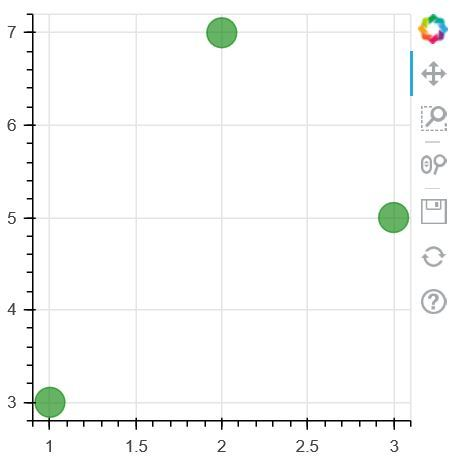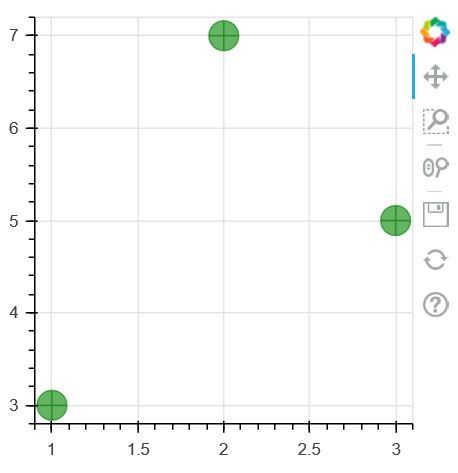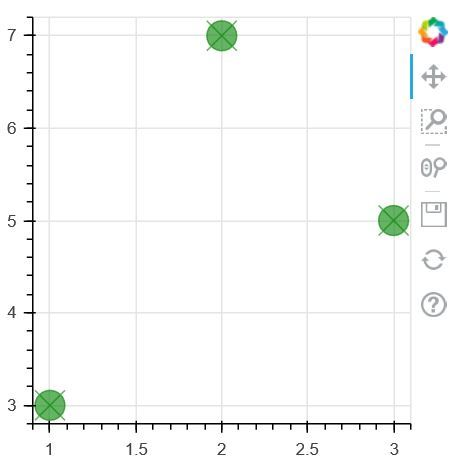# Make an Circle Glyphs in Python using Bokeh

Bokeh is a Python interactive data visualization. Unlike Matplotlib and Seaborn, Bokeh renders its plots using HTML and JavaScript. It targets modern web browsers for presentation providing elegant, concise construction of novel graphics with high-performance interactivity.

## Plotting the Circle Glyphs

Different shapes such as circle, rectangle, polygon, etc. can, be drawn by the figure object. Bokeh Figure class following methods to draw circle glyphs which are given below:

• circle()
• circle_cross()
• circle_x()

1. circle() method: circle() method is a used to add a circle glyph to the figure and needs x and y coordinates of its center.

Parameter:This method accept the following parameters that are described below:

• x: This parameter is the x-coordinates for the center of the markers.
• y: This parameter is the y-coordinates for the center of the markers.
• angle: This parameter is the angles to rotate the markers.
• fill_alpha: This parameter is the fill alpha values for the markers.
• fill_color: This parameter is the fill color values for the markers.
• radius: This parameter is the radius values for circle markers .
• radius_dimension: This parameter is the dimension to measure circle radii along.
• size: This parameter is the size (diameter) values for the markers in screen space units.

Example:

 `# Implementation of bokeh function  ` `    `  `import` `numpy as np   ` `from` `bokeh.plotting ``import` `figure, output_file, show  ` `    `  `plot ``=` `figure(plot_width ``=` `300``, plot_height ``=` `300``)  ` `plot.circle(x ``=` `[``1``, ``2``, ``3``], y ``=` `[``3``, ``7``, ``5``],   ` `            ``size ``=` `20``, color ``=``"green"``, alpha ``=` `0.6``)  ` `    `  `show(plot)  `

Output:2. circle_cross() method: circle_cross() method is a used to add a circle glyph with a ‘+’ cross through the center to the figure and needs x and y coordinates of its center.

Syntax: circle_cross(x, y, size=4, angle=0.0, *, angle_units=’rad’, fill_alpha=1.0, fill_color=’gray’, line_alpha=1.0, line_cap=’butt’, line_color=’black’, line_dash=[], line_dash_offset=0, line_join=’bevel’, line_width=1, name=None, tags=[], **kwargs)

Parameter:This method accept the following parameters that are described below:

• x: This parameter is the x-coordinates for the center of the markers.
• y: This parameter is the y-coordinates for the center of the markers.
• angle: This parameter is the angles to rotate the markers.
• fill_alpha: This parameter is the fill alpha values for the markers.
• fill_color: This parameter is the fill color values for the markers.
• radius: This parameter is the radius values for circle markers .
• radius_dimension: This parameter is the dimension to measure circle radii along.
• size: This parameter is the size (diameter) values for the markers in screen space units.

Example:

 `# Implementation of bokeh function  ` `     `  `import` `numpy as np   ` `from` `bokeh.plotting ``import` `figure, output_file, show  ` `     `  `plot ``=` `figure(plot_width ``=` `300``, plot_height ``=` `300``)  ` `plot.circle_cross(x ``=` `[``1``, ``2``, ``3``], y ``=` `[``3``, ``7``, ``5``],  ` `          ``size ``=` `20``, color ``=``"green"``, alpha ``=` `0.6``)  ` `     `  `show(plot)  `

Output:3. circle_x() method: circle_x() method is a used to add a circle glyph with a ‘X’ cross through the center. to the figure and needs x and y coordinates of its center.

Syntax: circle_x(x, y, size=4, angle=0.0, *, angle_units=’rad’, fill_alpha=1.0, fill_color=’gray’, line_alpha=1.0, line_cap=’butt’, line_color=’black’, line_dash=[], line_dash_offset=0, line_join=’bevel’, line_width=1, name=None, tags=[], **kwargs)

Parameter:This method accept the following parameters that are described below:

• x: This parameter is the x-coordinates for the center of the markers.
• y: This parameter is the y-coordinates for the center of the markers.
• angle: This parameter is the angles to rotate the markers.
• fill_alpha: This parameter is the fill alpha values for the markers.
• fill_color: This parameter is the fill color values for the markers.
• radius: This parameter is the radius values for circle markers .
• radius_dimension: This parameter is the dimension to measure circle radii along.
• size: This parameter is the size (diameter) values for the markers in screen space units.

Example:

 `# Implementation of bokeh function  ` `     `  `import` `numpy as np   ` `from` `bokeh.plotting ``import` `figure, output_file, show  ` `     `  `plot ``=` `figure(plot_width ``=` `300``, plot_height ``=` `300``)  ` `plot.circle_x(x ``=` `[``1``, ``2``, ``3``], y ``=` `[``3``, ``7``, ``5``], size ``=` `20``,  ` `         ``color ``=``"green"``, alpha ``=` `0.6``)  ` `     `  `show(plot)  `

Output:Whether you're preparing for your first job interview or aiming to upskill in this ever-evolving tech landscape, GeeksforGeeks Courses are your key to success. We provide top-quality content at affordable prices, all geared towards accelerating your growth in a time-bound manner. Join the millions we've already empowered, and we're here to do the same for you. Don't miss out - check it out now!